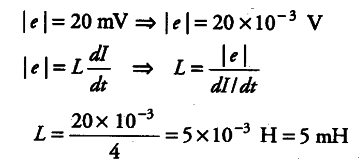# If a rate of change of current

If a rate of change of current of 4 A induces an emf of 20 mV in a solenoid, what is the self-inductance of the solenoid?

Here dI/dt = 4 A\${{s}^{-1}}\$